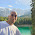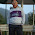# Modulo or Remainder Operator in Java

Modulo Operator is one of the fundamental operators in Java. It's a binary operator i.e. it requires two operands. In a division operation, the remainder is returned by using modulo operator. It is denoted by % (percentage) sign. For example 5%2 will return 1 because if you divide 5 with 2, the remainder will be 1. For a programmer it's very important to know how to use this operator, they are very important to build logic. For example, in many cases like reversing a number or checking if a number is a palindrome, you can use modulus operator with 10 to get the last digit, for example, 101%10 will return 1 or 1234%10 will return 4, the last digit. It is one of a rather less used arithmetic operators in comparison of +, -, * and /.  One of the important points about the remainder operator which is not known by many Java programmer is that it can also be used with floating point numbers.

It's surprising because you don't normally think of real number division as producing remainders. However, there are sometimes when it's useful to ask exactly how many times does 2.5 go into 7.5 and what's left over? The answer is that 2.5 goes into 7.5 three times with zero leftovers, and it's that zero which is the result of 7.5 % 2.5 in Java.

Another good use of modulus operator is to check if a number is even or odd. In case of even number, number%2 will return 0 while if a number is odd then number%2 will return 1.

## What is Modulo or Remainder Operator in Java

Modulo operator is also known as Remainder operator and denoted by percentage sign (%). It's one of the most basic operator and very useful to create logic in programming, available in almost every single programming language. As we learn in first paragraph, in Java modulus, operator can also be applied to floating point numbers e.g. 3.0%1.0 is perfectly legal in Java. Since it return remainder value in division operation it is also known as remainder operator.

If both operands for %, the modulus operator have type int, then operand_left %  operand_right evaluates to the integer remainder. For example, 11 % 3 evaluates to 2 because 11 divided by 3 has a remainder of 2.  Also if  both operands have type int, the modulus operator (with both operands) evaluates to int. If either or both operands of the mod operator have type double, then evaluating it produces the remainder.

This kind of mod operator does not exist in C or C++ where the mod operator only works with int operands. The evaluated result is a double value. For example, 8.27 % 2 evaluates to 0.27 since the division is 4 with a remainder of 0.27. Of course, both values can also be double, so 6.7 % 2.1 evaluates to 0.4 since the division is 3 with a remainder of 0.4. The remainder is computed by assuming the division yields an integer result, with the rest being a remainder

## How to use Modulo Operator in Java with Example

You cannot understand anything better without any example. Same is true for modulo operator in Java. In this example we shall show you how to use the modulo operator. The modulo operator is an arithmetic operator that is used to divide one operand by another and return the remainder as its result. You use the modulo operator to get the remainder of the division between an int variable and 10 and a double variable and 10, as described in the code snippet below.

```import java.util.Arrays;

/**
* Java program to demonstrate how to use modulo operator in Java. Modulo
* operator returns remainder of division operation between two operands. It's
* part of Java's arithmetic operator suite.
*
* @author Javin Paul
*/
public class Modulo{

public static void main(String args[]) {

// Example 1 - Modulo operator returns remainder
int iValue = 101;
double dValue = 39.02;

System.out.println(iValue + " mod 9 = " + iValue % 9);
System.out.println(dValue + " mod 9 = " + dValue % 9);

// Example 2 - module operator on 10 can give you last digit of integer number
int number = 215;
int total = 349;
System.out.printf("Last digit of %d is %d%n", number, number % 10);
System.out.printf("Last digit of %d is %d%n", total, total % 10);

// Example 3 - You can use modulo operator on 2 to check if number is even or odd
int even = 22;
int odd = 21;
System.out.printf("%d is %s number%n", even, oddness(even));
System.out.printf("%d is %s number%n", odd, oddness(odd));

}

public static String oddness(int i) {
return i % 2 == 0 ? "even" : "odd";
}

}

Output :
101 mod 9 = 2
39.02 mod 9 = 3.020000000000003
Last digit of 215 is 5
Last digit of 349 is 9
22 is even number
21 is odd number```

## Important points about Modulo Operator in Java

Now we know what is modulus or remainder operator, let's revisit some important things about this useful operator :

1) It is also known as remainder operator and denoted by percentage sign %
2) You can use remainder operator to get the last digit of any number e.g. number%10 will give you last digit, a useful trick to solve many numeric problems.
3) modulus operator is not just applicable to integral types e.g. byte, short, int, long but also to floating point types e.g. float and double.
4) You can also use remainder operator to check if a number is ever or odd, or if a year is leap year.

That's all about how to use modulo operator in Java. As I said, its one of the important operator and can be very handy in both real world development as well as solving coding questions during interviews.

Further Learning
Complete Java Masterclass
Java Fundamentals: The Java Language
Java In-Depth: Become a Complete Java Engineer!

1.Can we use modulo operator with float or double in Java also?

2.One of the most important thing to remember about modulo operator in Java is how it works with negative operands. For example, what do you think following operations will print :

int mod = -20 % 3;
System.out.println("value of -20 % 3 = " + mod);

mod = 20 % (-3);
System.out.println("value of 20 % (-3) = " + mod);

mod = -20 % (-3);
System.out.println("value of -20 % (-3) = " + mod);

when you run these snippets, you will get something like
value of -20 % 3 = -2
value of 20 % (-3) = 2
value of -20 % (-3) = -2

do you understand why? as many of you might have expected +2 in last operation and -2 in second operation.
well, Java's modulo or mod or remainder operator uses sign of dividend (top operand) and not of divisor (bottom operand).

Which means, if dividend is negative then remainder will be negative and if dividend is positive then result would be positive, irrespective of sign of divisor. this also explains the output, because you get negative remainder in first case because dividend was negative, second result was positive again because dividend was positive and third result is negative irrespective of divisor is also negative. Remember this concept, if you are going for SCJP or OCAJP, many programmer get it wrong.

1.What about the other way around, when the dividend is smaller than the divisor?
int result = (-5) % 3; // gives -2.
int result = (-3) % 5; // gives -3.
In general, int result = (-a) % b; gives the right answer when |-a| > b.
In order to get the proper result when |-a| < b we should wrap the divisor.
int result = ((-a) % b) + b; for negative a or int result = (((-a) % b) + b) % b; for positive or negative a
http://docs.oracle.com/javase/specs/jls/se8/html/jls-15.html#jls-15.17.3

3.Though you have already listed couple of interesting examples of remainder operator in Java, I would like to add few more :
1) to check if number is even or odd, if(number%2) == 0 then number is even
2) get the last digit in any base, for example in decimal, number%10 will return last digit, in binary number%2 will return last bit, in octal number%8 will return last octat and in hexadecmial number%16 should return last digit.
3) You can use remainder operator to get every nth count e.g.
if ( everyFiftCustomer++ % 5 == 0 ) {
giveDiscount();
}

4) You can check if an year is leap year or not by using following logic
if (((year % 4 == 0) && (year % 100 != 0)) || (year % 400 == 0)
leap year
else
not a leap year

5) Used in circular array and hashtable to conain elements in limit.

4.Thanks for listing these interesting use of modulus operator. One more I can add is on implementing hash function on hash table to convert hash keys into array indexes. For example, if your hash table is backed by an array or size 10, and you want to store arbitrary integers on this table, you need to convert those numbers into valid array index e.g. 0 to 9. We can use modulus operaotr here.

for example, if you want to store 56 in this table, 56%9 will give you 2, so you can store 56 there. When you want it back, use the same hash function again.

1.@Anonymous, good point, modulo operator was indeed the simplest of hash function

5.If user enters a digit With o at end like 20,30 then how will the use of mod give the last digit

1.Hello @Subhrajit, 20%10 will return zero and that's your last digit, isn't it?

6.public static void main(String[] args) {
// TODO code application logic here
int n,dec=0,i=0,N=0;
Scanner in=new Scanner(System.in);
System.out.println("Enter number in binary form like 0 1 or 1 0");
n=in.nextInt();
while(n > 0)

{
dec = n % 10;
N =N+ dec *( int)Math.pow(2,i);
i++;
n=n/10;
}
System.out.println("Decimal \t"+N);
}
}
Can you explain me the dry run of above program when binay is 11.